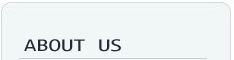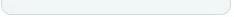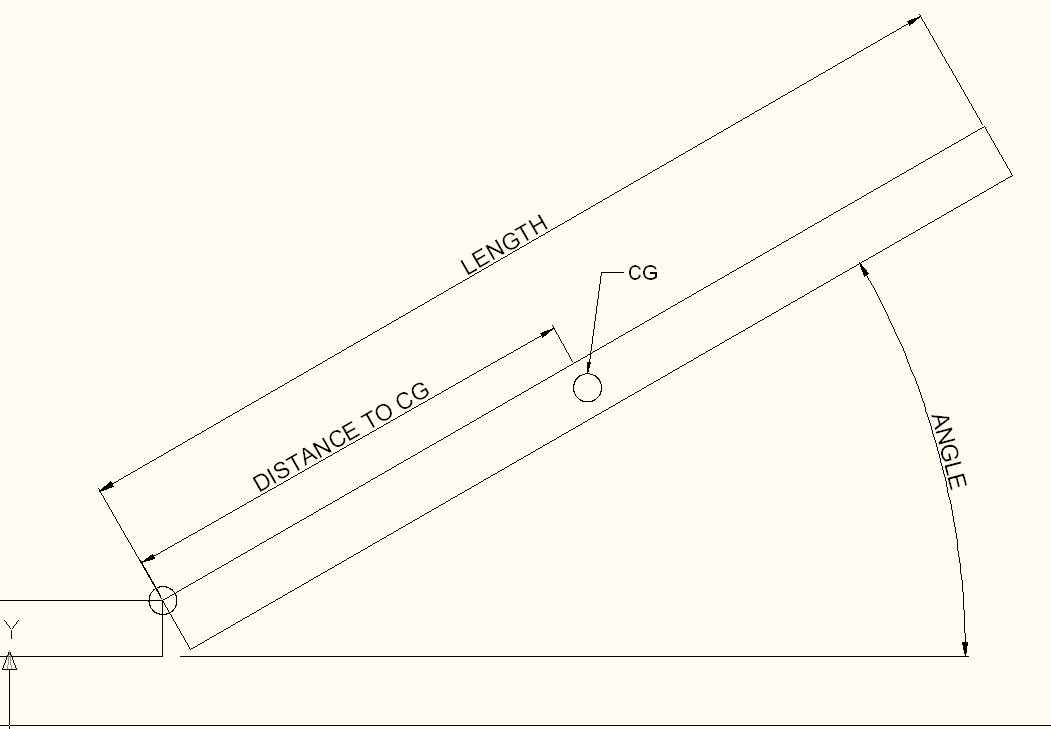﻿ Friction hinge, Custom hinge, Laptop hinge, Monitor hinge, torque hinge, LCD hinge, Furniture hinge, Medical hinge, oven hinge | Shinelot Enterprise Co.,About Us Our philosophy Hinge Features Torque Calculation F & QTorque CalculationTorque=Weight(display)xDistance to Center of Gravity x Cosine(Angle)   . Distance to CG is 1/2 of the length if the weight is evenly distributed   . The angle is the most torque required to hold, otherwise, please assume zero degree    Example:  Weight=3 lb  Length=10 inch  Distance to Center of gravity=5 inch(evenly distributed)  Angle: 45 degree  Torque=(3lb)x(5in)x(cos45)=10.61 lb.in